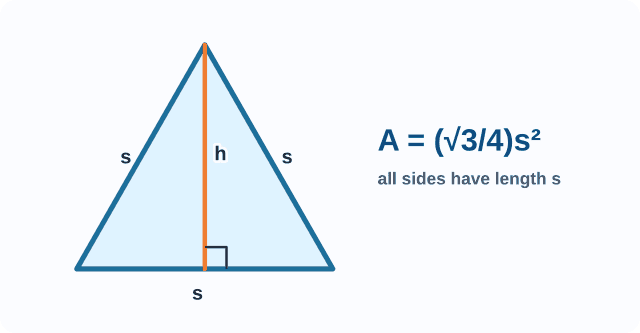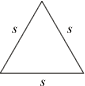# equilateral triangle

Equilateral Triangle

A triangle with three congruentsides.

Note: An equilateral triangle is also equiangular. In Euclidean geometry, the angles of an equilateral triangle each measure 60°.

 Equilateral Triangles = length of a side